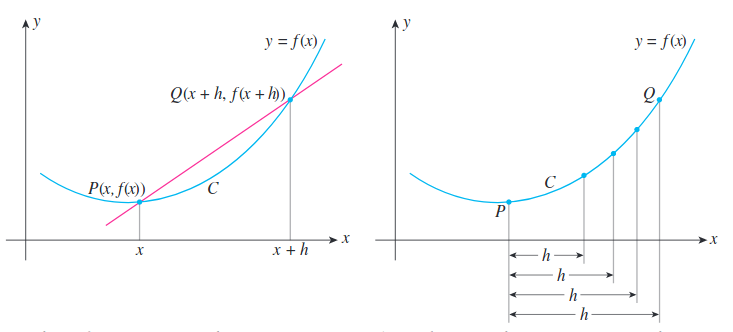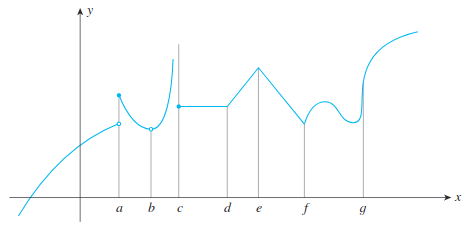### Model Preliminaries

Derivatives and functions

• The derivative a function is a measure of rate of change; it measures how much the value of function $f(x)$ changes when we change parameter $x$. Typically, we want to differentiate the dependent variables $f(x)$ or $y$, with respect to the independent variables.

• For single-variable functions, the derivative of $f$ with respect to $x$ is denoted by $f’(x)=\frac{df}{dx}(x)$, where the slope of the tangent line to the function $f$ at point $P(x, f(x))$ is given by:

\begin{equation} \frac{df}{dx}(x) = \lim_{h\to0}\frac{f(x+h)-f(x)}{h} \end{equation}Image taken from Applied Calculus for the Managerial, Life and Social Sciences 8th ed

• Without applying limits, the difference quotient $\frac{f(x+h)-f(x)}{h}$ measures the average (not point rate of change of $f(x)$ with respect to $x$ over the interval $[x, x+h]$.

• $\lim_{h\to0}$ is used to express $h$ which gets infinitely small but non-zero. Intuitively, this tells us what will happen to $f(x)$ when we add infinitely small values to $x$. (Limit notation is used because we cannot express as $h$ become 0 because division by 0 is undefined.)

• The derivative of a function $f$ is itself a function $f’$ that gives the slope of the tangent line(slope) to the graph of $f$ at any point $(x, f(x))$, which we can use to calculate rate of change.

• As derivatives measure rate of change and represent the slope of the function, they can be used to determine intervals or points where the function is increasing or decreasing. If the derivative $f’(x)$ is positive at $x$, it means the function is increasing at $x$. If the derivative is negative at $x$, it means the function is decreasing at $x$.

• In the context of machine learning, we search for parameter values, $x$ along a family of functions $f$. i.e. when fitting a function, we want to find the parameter values by changing $x$ until we get a derivative that is close to or equal to 0.

High-school refresher - In order to maximise or minimise a function, we can set its derivative equal to zero, and solve for the parameters. This was easy because our functions were linear in terms of the input variable. However in ML it is common to have non-linear relationships, and functions that have hundreds of dimensions and it is not analytically tractable to obtain the closed-form solution. Thus, we need an iterative algorithm to solve.

Differentiability and Loss functions

• In the real-world, we may encounter non-differentiable functions that do not have a derivative at certain values. These can occur for multiple reasons, primarily because there is a jump in $f(x)$, there is a sharp change in $f(x)$ as illustrated in the following:Image taken from Applied Calculus for the Managerial, Life and Social Sciences 8th ed

• In a binary classification setting, the empirical loss $(0-1)$ is given by $h(x)\neq f(x)$ thatwhich is non-differentiable(and non-continuous). Thus we often use surrogate loss functions to make the errir differentiable with respect to the input parameters. Cross-entropy, mean-squared-error, logistic etc are functions that wrap around the true loss value to give a surrogate or approximate loss which is differentiable.

• This principle is also used when considering ‘smooth’ activation functions for neural networks and allows us to apply gradient descent.

• The significance of smoothness is that we can approximate it to be linear at different coordinate points.

Note that although the loss function might be convex, the problem as a whole can still be non-convex, and there is no guarantee that the parameter values will converge to a global optimum.

Applied Calculus for the managerial, life, and social sciences